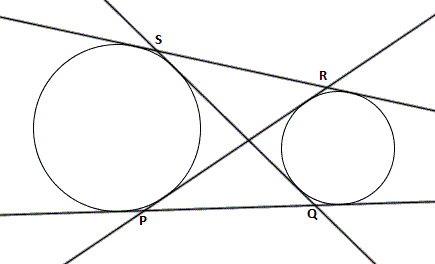# Do I Need A Scale?

Geometry Level 2In the above figure $SR = 6$, the radius of larger circle is $5$ and that of the smaller circle is $3$. Find the length of $PQ$.

×